Get ICSE Solutions for Class 10 Mathematics Chapter 17 Mensuration for ICSE Board Examinations on ICSESolutions.com. We provide step-by-step solutions for ICSE Mathematics Class 10 Solutions Pdf. You can download the Class 10 Maths ICSE Textbook Solutions with a Free PDF download option.

## Mensuration Class 10 Maths ICSE Solutions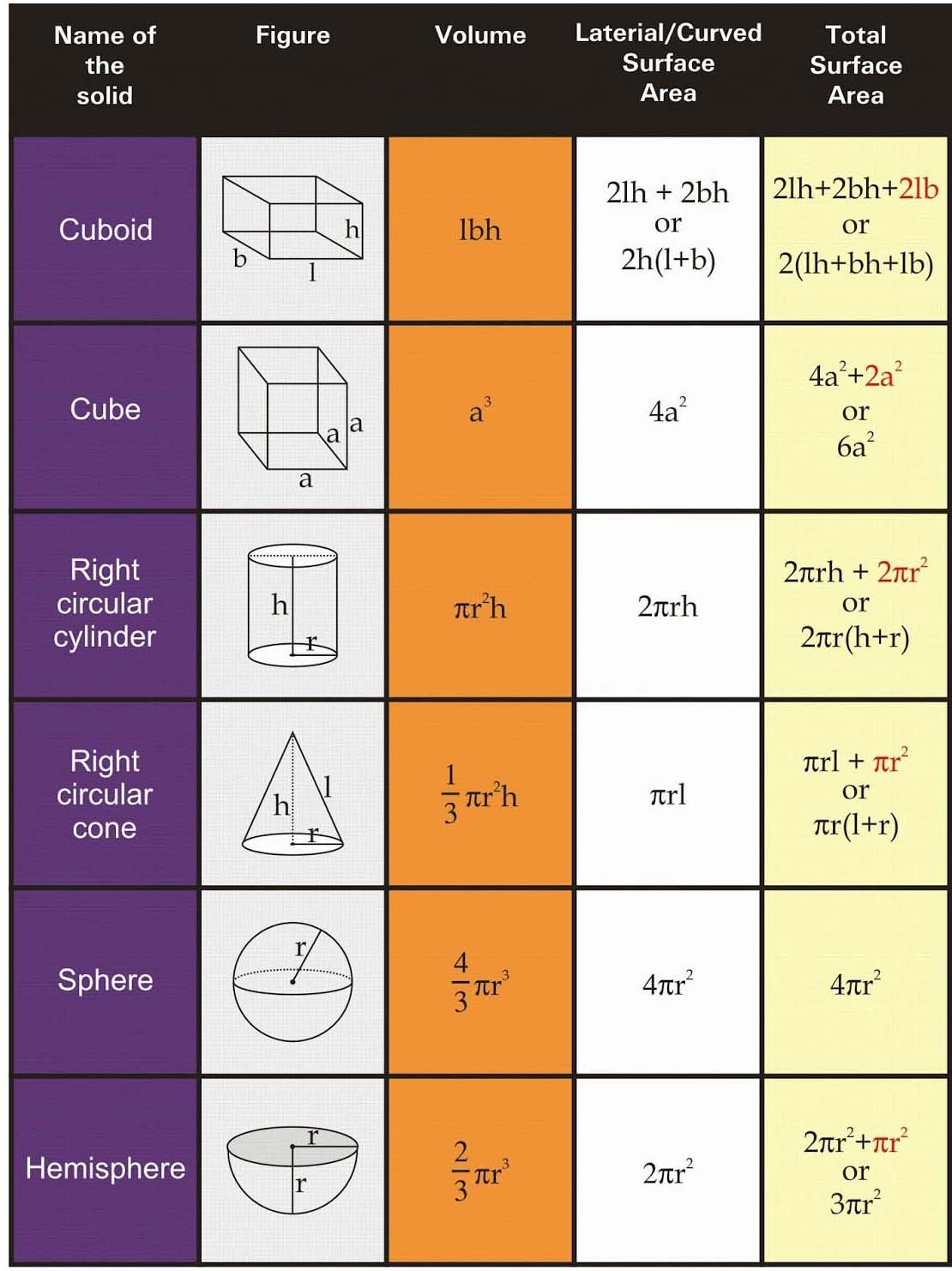### Formulae

Perimeter:

1. The perimeter of a plane figure = sum of lengths of its sides.
2. Circumference of a circle = 2πr,
where r is the radius of the circle.

Area (of Plane Figures):

1. Area of a triangle = ½ × base × height.
2. Area of a triangle (Heron’s formula)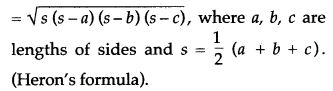3. Area of an equilateral triangle = $$\frac{\sqrt{3}}{4}{{a}^{2}}$$
where a is its side.
4. Area of an isosceles triangle5. Area of a quadrilateral (when diagonals intersect at right angles) = ½ × product of diagonals.
6. Area of a rectangle = length × breadth.
7. Area of a square = (side)2.
8. Area of a parallelogram = base × height.
9. Area of a rhombus = ½ × product of diagonals.
10. Area of a trapezium = ½ × (sum of parallel sides) × height.
11. Area of a circle = πr2 where r is the radius of the circle.
12. Area of a circular ring = π (R2 – r2)
where R and r are the radii of the outer and the inner circles.

Surface Area and Volume of Solids:

1. Cube Cuboid:
(i) Surface area of a cube = 6a2
where a is its edge (side).
(ii) Surface area of a cuboid = 2 (ℓb + bh + ℓh)
where ℓ, b and h are its edges.
(iii) Surface area of four walls (lateral surface area) of a cuboid = 2h (ℓ + b)
where ℓ, b and h are its edges.
(iv) Volume of a cube = (side)3.
(v) Volume of a cuboid = length × breadth × height.
2. Solid Cylinder:
Let r and h be the radius and height of a solid cylinder, then
(i) Curved (lateral) surface area = 2πrh.
(ii) Total surface area = 2πr (h + r).
(iii) Volume = πr2h.
3. Hollow Cylinder:
Let R and r be the external and internal radii, and h be the height of a hollow cylinder, then
(i) External curved surface area = 2πRh.
(ii) Internal curved surface area = 2πrh.
(iii) Total surface area = 2π (Rh + rh + R2 – r2).
(iv) Volume of material = π (R2 – r2)h.
4. Cone: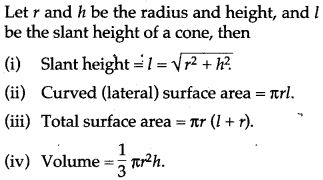5. Solid Sphere: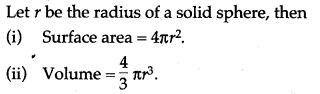6. Spherical Shell:7. Solid Hemisphere: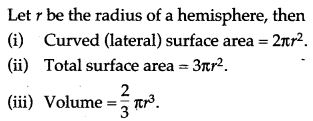8. Hemispherical Shell: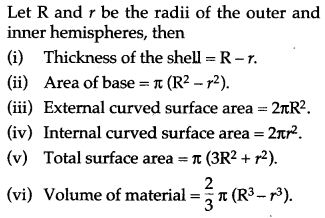### Formulae Based Questions

Question 1. Find the area of a circle whose circumference is 22 cm.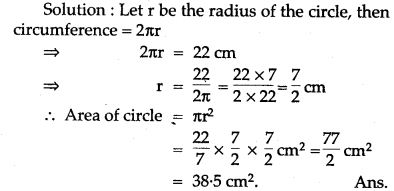Question 2. If the perimeter of a semi-circular protractor is 36 cm. Find its diameter.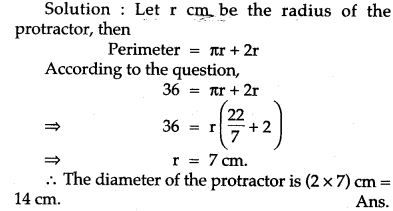Question 3. A well 28.8 m deep and of diameter 2 m is dug up. The soil dug out is spread all around the well to make a platform 1 m high considering the fact lose soil settled to a height in the ratio 6 : 5 find the width of the platform.Question 4. Two-cylinder have bases of the same size. The diameter of each is 14 cm. One of the cones is 10 cm high and the other is 20 cm high. Find the ratio between their volumes.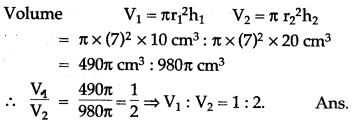Question 5. A glass cylinder with a diameter of 20 cm water to a height of 9 cm. A metal cube of 8 cm edge is immersed in it completely. Calculate the height by which water will rise in the cylinder. (Take π = 3.142)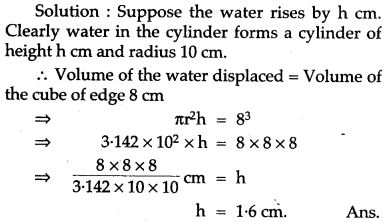Question 6. Water is being pumped out through a circular pipe whose external diameter is 7 cm. If the flow of water is 72 cm per second how many liters of water are being pumped out in one hour.Question 7. The diameter of a garden roller is 1.4 m and it is 2 m long. How much area will it cover in 5 revolutions? (Take π = 22/7)Question 8. The radius and height of a cylinder are in the ratio of 5 : 7 and its volume is 550 cm. Find its radius. (Take π = 22/7)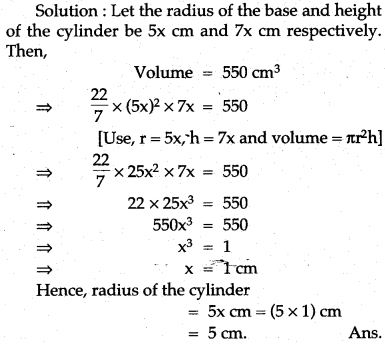Question 9. The ratio of the base area and curved surface of a conical tent is 40 : 41. If the height is 18 m, Find the air capacity of the tent in terms of n.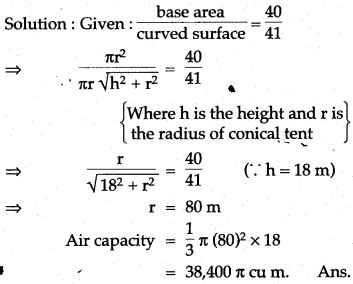Question 10. The diameter of two cones are equal. If their slant heights be in the ratio of 5 : 4. Find the ratio of their curved surface areas?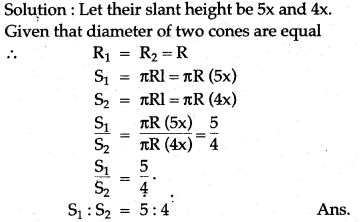Question 11. The radius and height of cone are in the ratio 3 : 4. If its volume is 301.44 cm3. What is its radius? What is its slant height? (Take π = 3.14)Question 12. Find the volume and surface area of a sphere of diameter 21 cm.Question 13. The volume of a sphere is 905 1/7 cm3, find its diameter.Question 14. There is surface area.and volume of sphere equal, find the radius of sphere.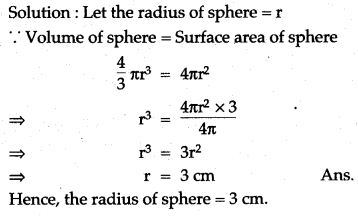Question 15. There is a ratio 1 : 4 between surface area of two spheres, find the ratio between their radius.Question 16. Marbles of diameter 1.4 cm are dropped into a beaker containing some water are fully submerged. The diameter of beaker is 7 cm. Find how many marbles have been dropped in it if the water rises by 5.6 cm.Question 17. Aspherical cannonball, 28 cm in diameter is melted and recast into a right circular conical mould, the base of which is 35 cm in diameter. Find the height of the cone, correct to one place of decimal.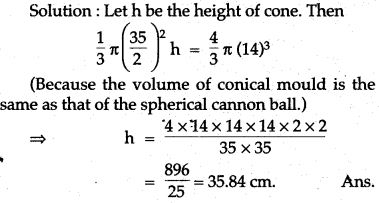Question 18. A cone and a hemisphere have equal bases and equal volumes. Find the ratio of their heights.Question 19. A solid sphere of radius 15 cm is melted and recast into solid right circular cones of radius 2.5 cm and height 8 cm. Calculate the number of cones recast.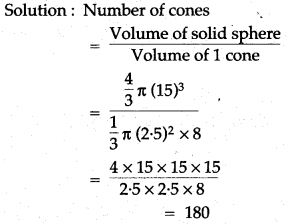Question 20. The radius of two spheres are in the ratio of 1 : 3. Find the ratio between their volume.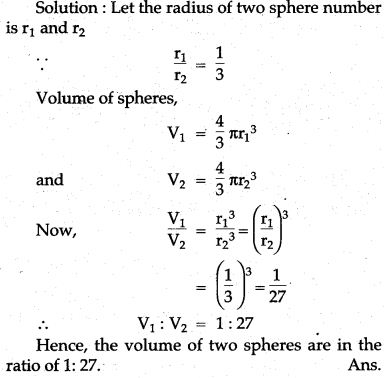Question 21. A hollow sphere of internal and external radii 6 cm and 8 cm respectively is melted and recast into small cones of base radius 2 cm and height 8 cm. Find the number of cones.Question 22. A sphere cut out from a side of 7 cm cubes. Find the volume of this sphere?Question 23. How many spherical bullets can be made out of a solid cube of lead whose edge measures 44 cm, each bullet being 4 cm in diameter?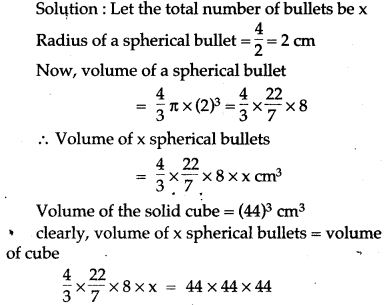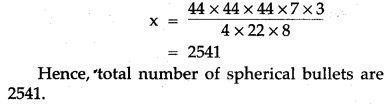Question 24. A hemispherical bowl of diameter 7.2 cm is filled completely with chocolate sauce. This sauce is poured into an inverted cone of radius 4.8 cm. Find the height of the cone.Question 25. The total surface area of a hollow metal cylinder, open at both ends of external radius 8 cm and height 10 cm is 338π cm2. Taking r to be inner radius, write down an equation in r and use it to state the thickness of the metal in the cylinder.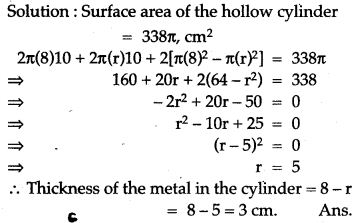Question 26. Find the weight of a lead pipe 35 cm long. The external diameter of the pipe is 2.4 cm and thickness of the pipe is 2mm, given 1 cm3 of lead weighs 10 gm.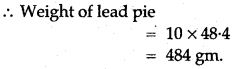Question 27. A glass cylinder with diameter 20 cm has water to a height of 9 cm. A metal cube of 8 cm edge is immersed in it completely. Calculate the height by which the water will up in the cylinder. Answer correct of the nearest mm. (Take π = 3.142)Question 28. A road roller is cylindrical in shape, its circular end has a diameter of 1.4 m and its width is 4 m. It is used to level a play ground measuring 70 m × 40 m. Find the minimum number of complete revolutions that the roller must take in order to cover the entire ground once.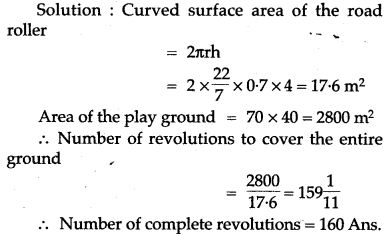Question 29. A vessel is in he form of an inverted cone. Its height is 11 cm., and the radius of its top which is open, is 2.5 cm. It is filled with water up to the rim. When lead shots, each of which is a sphere of radius 0.25 cm., are dropped 2 into the vessel, 2/5 th of the water flows out. Find the number of lead shots dropped into the vessel.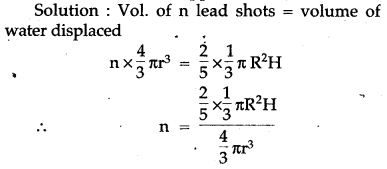### Prove the Following

Question 1. The circumference of the base of a 10 m high conical tent is 44 metres. Calculate the length of canvas used in making the tent if width of canvas is 2m. (Take π = 22/7)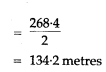### Figure Based Questions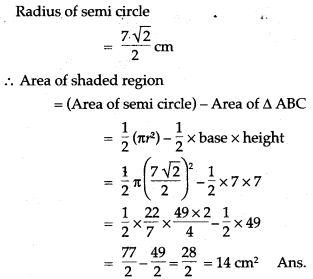Question 3. In the adjoining figure, the radius is 3.5 cm. Find:
(i) The area of the quarter of the circle correct to one decimal place.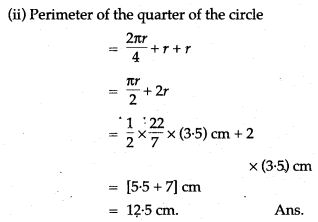Question 4. The boundary of the shaded region in the given diagram consists of three semicircular areas, the smaller ones being equal and it’s diameter 5 cm, if the diameter of the larger one is 10 cm, calculate:Question 5. In the given figure, AB is the diameter of a circle with centre O and OA = 7 cm. Find the area of the shaded region.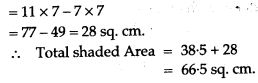Question 6. Find the perimeter and area of the shaded portion of the following diagram; give your answer correct to 3 significant figures. (Take π = 22/7).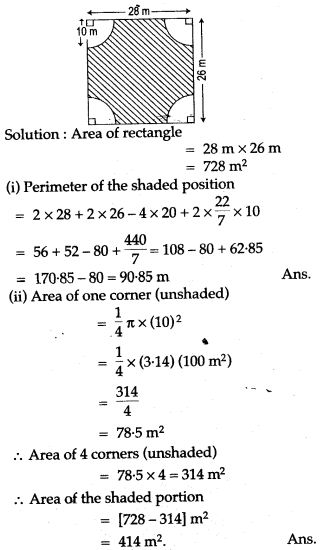Question 7. In the adjoining figure, crescent is formed by two circles which touch at the point A. O is the centre of bigger circle. If CB = 9 cm and DE = 5 cm, find the area of the shaded portion.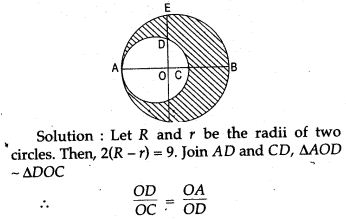Question 8. In the given figure, find the area of the unshaded portion within the rectangle. (Take π = 22/7).Question 9. The figure shows a running track surrounding a grassed enclosure PQRSTU. The enclosure consists of a rectangle PQST with a semicircular region at each end, PQ = 200 m; PT = 70 meter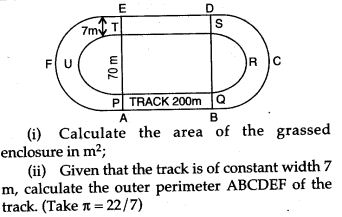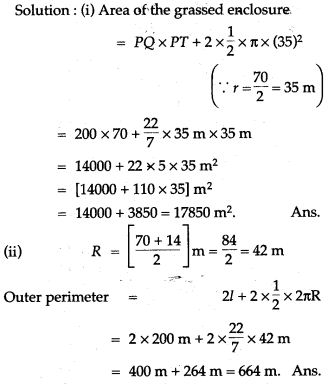Question 10. A doorway is decorated as shown in the figure. There are four semi-circles. BC, the diameter of the larger semi-circle is of length 84 cm. Centres of the three equal semi-circles lie on BC. ABC is an isosceles triangle with AB = AC. If BO = OC, find the area of the shaded region. (Take π = 22/7).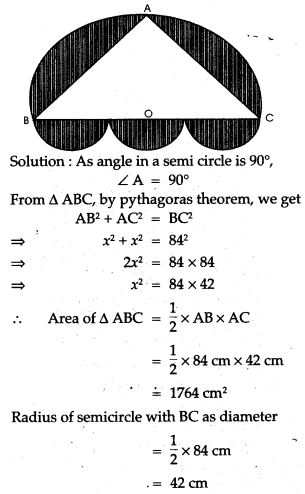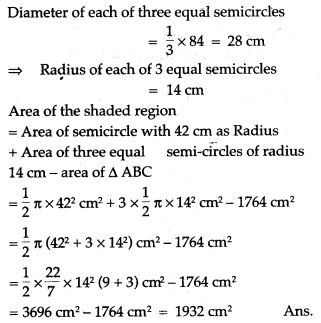Question 11. In the figure given below, ABCD is a rectangle. AB 14 cm, BC = 7 cm. From the rectangle, a quarter circle BFEC and a semicircle DGE are removed. Calculate the area of the remaining piece of the rectangle. (Take π = 22/7).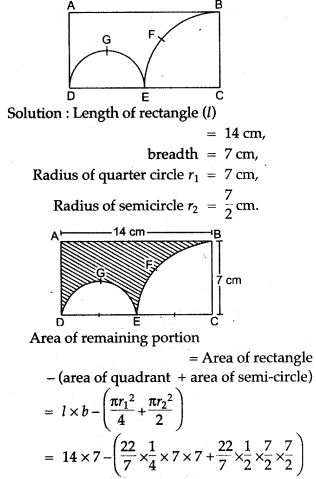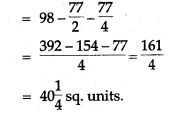Question 12. The given figure represents a hemisphere surmounted by a conical block of wood. The diameter of their bases is 6 cm each and the slant height of the cone is 5 cm. Calculate:
(i) the height of the cone.
(ii) the vol. of the solid.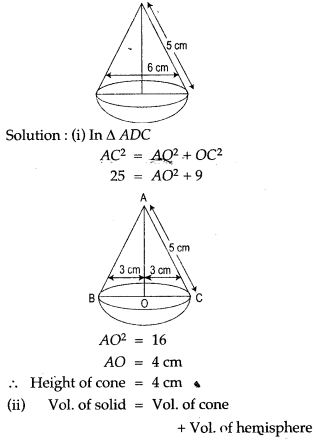Question 13. Calculate the area of the shaded region, if the diameter of the semi circle is equal to 14 cm. (Take π = 22/7).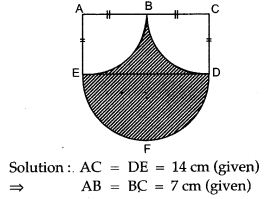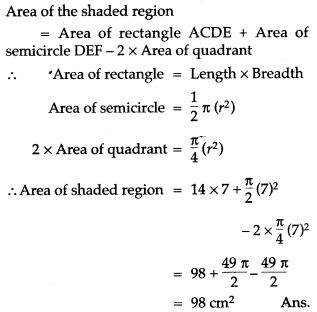Question 14. With reference to the figure given a alongside, a metal container in the form of a cylinder is surmounted by a hemisphere of the same radius. The internal height of the cylinder is 7 m and the internal radius is 3.5 m. Calculate: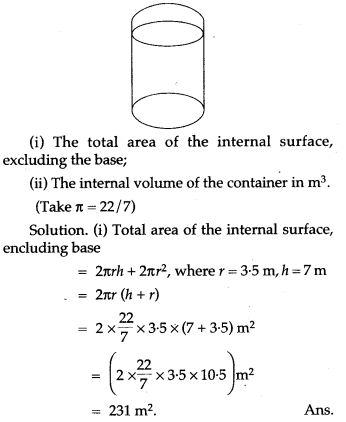### Concept Based Questions

Question 1. A bucket is raised from a well by means of a rope which is wound round a wheel of diameter 77 cm. Given that the ascends in 1 minute 28 seconds with a uniform speed of 1.1 m/sec, calculate the number of complete revolutions the wheel makes in raising the bucket. (Take π =22/7)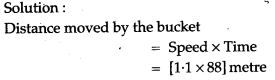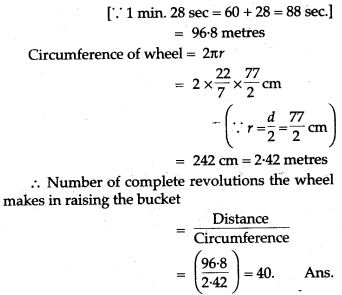Qustion 2. The radius of two right circular cylinder are in the ratio of 2 : 3 and their heights are in the ratio of 5 : 4 calculate the ratio of their curved surface areas and also the ratio of their volumes.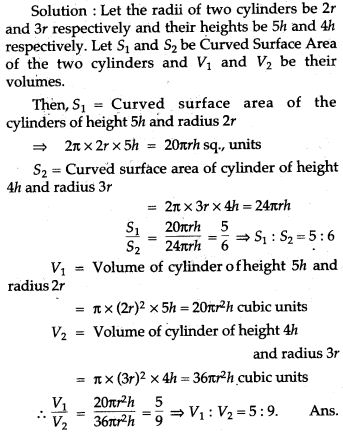Question 3. A vessel in the form of an inverted cone is filled with water to the brim: Its height is 20 cm and diameter is 16.8 cm. Two equal solid cones are dropped in its so that they are fully submerged. As a result, one third of the water in the original cone overflows. What is the volume of each of the solid cones submerged?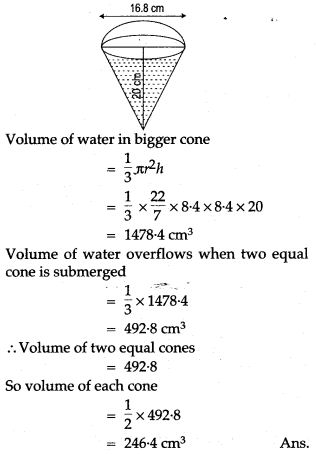Question 4. Water flows at the rate of 10 m per minute through a cylindrical pipe 5 mm of diameter. How much time would it take to fill a conical vessel whose diameter at he surface is 40 cm and depth is 24 cm?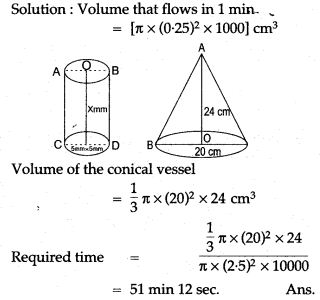Question 5. A conical tent is accommodate to 11 persons each person must have 4 sq. metre of the space on the ground and 20 cubic metre of air to breath. Find the height of the cone.Question 6. A circus tent is cylindrical to a height of 3 meters and conical above it. If its diameter is 105 m and the slant height of the conical portion is 53 m calculate the length of the canvas which is 5m wide to make the required tent.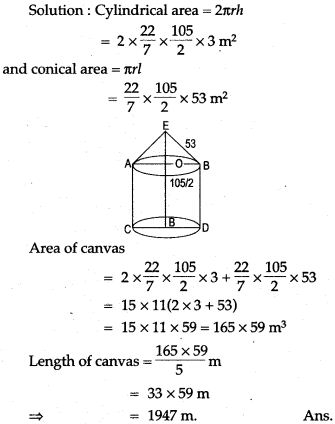Question 7. An exhibition tent is in the form of a cylinder surmounted by a cone. The height of the tent above the ground is 85 m and the height of the cylindrical part is 50m. If the diameter of the base is 168 m, find the quantity of canvas required to make the tent. Allow 20% extra for folds’ and for stitching. Give your answer to the nearest m2.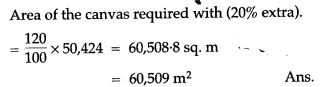Question 8. The radius of a sphere is 10 cm. If we increase the radius 5% then how many % will increase in volume?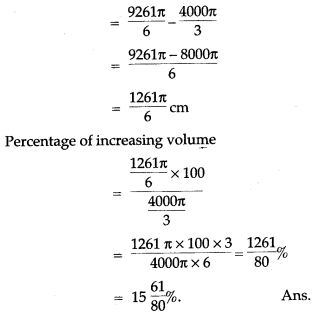Question 9. The cylinder of radius 12 cm have filled the 20 cm with water. One piece of iron drop in the stands of water goes up 6.75 cm. Find the radius of sphere piece.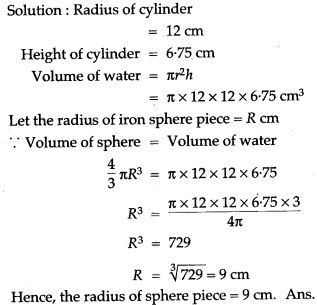Question 10. The radius of the internal and external surfaces of a hollow spherical shell are 3 cm and 5 cm respectively! If it is melted and recast into a solid cylinder of height 8/3 cm, find the diameter of the cylinder.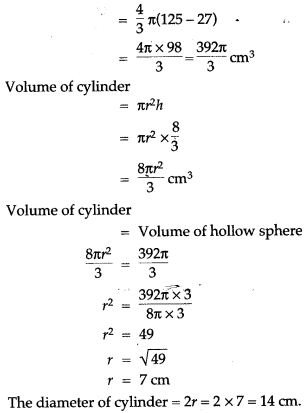Question 11. The surface area of a solid metallic sphere is 616 cm2. It is melted and recast into smaller spheres of diameter 3.5 cm. How many such spheres can be obtained?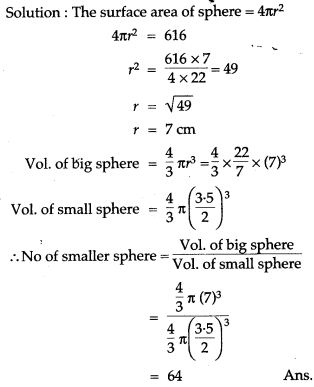Question 12. The diameter of the cross section of a water pipe is 5 cm. Water flows through it at 10km/hr into a cistern in the form of a cylinder. If the radius of the base of the cistern is 2.5 m, find the height to which the water will rise in the cistern in 24 minutes.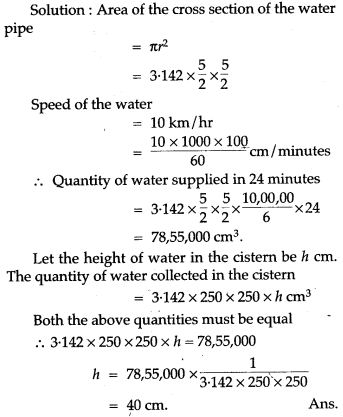Question 13. A metallic cylinder has radius 3 cm and height 5 cm. It is made of metal A. To reduce its weight, a conical hole is drilled in the cylinder, as shown and it is completely filled with a lighter metal B. The conical hole has a radius of 3/2 cm and its depth is 8/9 cm. Calculate the ratio of the volume of the metal A to the volume of the metal B in the solid.Question 14. An iron pillar has some part in the form of a right circular cylinder and remaining in the form of a right circular cone. The radius of the base of each of cone and cylinder is 8 cm. The cylindrical part is 240 cm high and the conical part is 36 cm high. Find the weight of the pillar if one cubic cm of iron weight is 7.8 grams.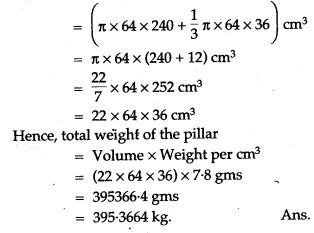Question 15. A spherical ball of radius 3 cm is melted and recast into three spherical balls. The radii of two of the balls are 1.5 cm and 2 cm. Find the diameter of the third ball.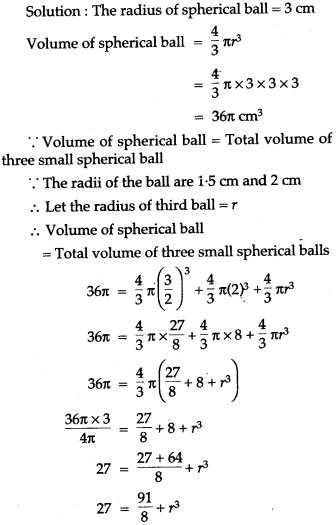Question 16. A buoy is made in the form of a hemisphere surmounted by a right cone whose circular base coincides with the plane surface of hemisphere. The radius of the base of the cone is 3.5 metres and its volume is two thirds of the hemisphere. Calculate the height of the cone and the surface area of buoy correct to two places of decimal.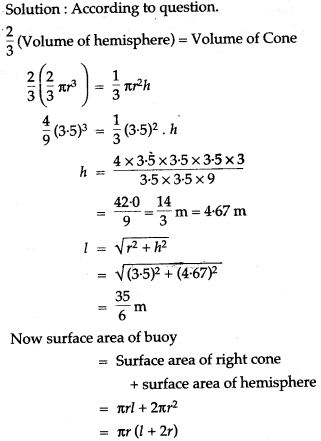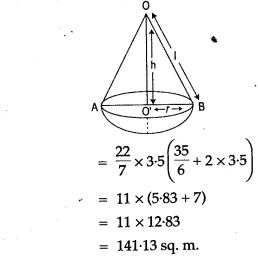Question 17. Water flows through a cylindrical pipe of internal diameter 7 cm at 36 km/hr. Calculate the time in minutes it would take to fill cylindrical tank, the radius of whose base is 35 cm and height is 1 m.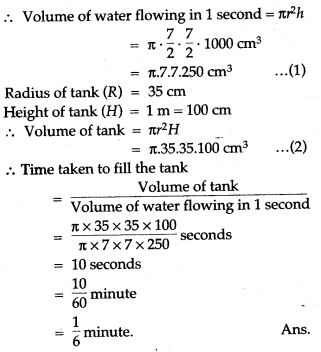For More Resources